Impact Factor 3.161 | CiteScore 3.13
More on impact ›

# Frontiers in Psychiatry

## Review ARTICLE

Front. Psychiatry, 08 October 2010 | https://doi.org/10.3389/fpsyt.2010.00127

# Age-dependent branching processes for surveillance of vaccine-preventable diseases with incubation period

• 1 Faculty of Mathematics and Informatics, Sofia University, Sofia, Bulgaria
• 2 Institute of Mathematics and Informatics, Bulgarian Academy of Sciences, Sofia, Bulgaria
• 3 Department of Mathematics, University of Extremadura, Badajoz, Spain

The purpose of this paper is to review the recent results of the authors in the area of infectious disease modeling by means of branching stochastic processes. This is a new approach involving age-dependent branching models, which turned out to be more appropriate and flexible for describing the spread of an infection in a given population, than discrete time ones. Concretely, Bellman–Harris and Sevast’yanov’s branching processes are investigated. It is justified that the proposed models are proper candidates as models of infectious diseases with incubation period like measles, mumps, avian flu, etc. It is worth to notice that in general the developed methodology is applicable to the diseases that follow the so-called SIR (susceptible–infected–removed) scheme in terms of epidemiological models. Two policies of extra-vaccination level are proposed and compared on the ground of simulation examples.

## Introduction

One of the main purposes of public health institutions is the surveillance and control of vaccine-preventable diseases. Common practice for such diseases is to observe and monitor the basic reproduction number, R0, and keeping it “well” below unity by means of additional vaccination of susceptible individuals into the population. How to determine this vaccination level is an important problem which depends on multiple factors. A significant factor for public authorities to assess the vaccination efficiency, is the time that the infectious disease should be allowed to survive after vaccination.

The research under survey in this paper deals with the problem of developing probabilistic methods to establish optimal vaccination levels based on controlling the time to extinction of the disease. Our aim is not only to keep R0 less than 1, but also that extinction of outbreaks occurs within a given period of time. To this end we model the spread of the infectious disease by way of branching processes.

Branching stochastic processes have been applied widely to model epidemic spread (see for example the monographs Daley and Gani, 1999; Andersson and Britton, 2000; Mode and Sleemam, 2000; Pakes, 2003). In terms of epidemic spreading we have drawn our attention to the SIR (susceptible–infective–removed) scheme. Measles, mumps or avian flu are examples of infectious diseases that follow this spreading scheme model. Let us notice that branching processes approach is appropriate for homogeneously mixing population, when the number of infected individuals is small in relation to the total population size (see Isham, 2005). For that reason, we assume this scenario, which would clearly correspond to the early stages of an epidemic.

The study of the spread of an infectious disease following the SIR model and depending on a vaccination level has been developed by De Serres et al. (2000) using branching processes in discrete time. However, these models are not efficient enough when trying to consider time to extinction in real time. This led us to the idea of our original paper (see González et al., 2010a) to suggest a more accurate approach to this problem by modeling the spread of the disease by continuous-time branching processes. Specifically, we have proposed to model the number of infectious individuals in the population depending on the vaccination level by means of Bellman–Harris branching processes (BHBP). To further elucidate the method of our modeling and to make the model more realistic, later on we have considered Sevast’yanov’s branching processes (SBP; see González et al., 2010b), which actually generalize BHBP. Both kinds of branching processes are particular cases of the general branching process (see Jagers, 1975), also called Crump–Mode–Jagers branching process, which is the most adequate model to fit infectious diseases following SIR scheme (see Ball and Donnelly, 1995). However, the advantage of SBP is that it gives us a more simplified framework being especially suitable to model the evolution of infectious diseases with incubation period (and a negligible contact period in comparison with it) for which the virulence of the disease could be a function of the length of this period.

For such kind of diseases, our main target was to determine the optimal proportion of susceptible individuals, which might be extra vaccinated to guarantee the extinction of the disease within a given period of time. To this end, firstly we established stochastic monotonicity and continuity properties of the distribution of the time that the infection survives, depending on the vaccination coverage rate, based on both BHBP and SBP (see González et al., 2009; González et al., 2010a). In this paper we review the main results obtained on this topic. Specifically, in Section “Branching Model of Epidemic Spread”, we introduce the BHBP and SBP in the epidemic context and provide their main properties. In Section “Vaccination Policies”, we develop two methods of obtaining optimal coverage rate of susceptible individuals after the vaccination process ended, one based on the mean of the time to extinction and another one on the quantiles of its distribution. A comparison of these vaccination policies based on simulated examples is given in Section “Comparison of Vaccination Policies Based on Simulations”. The paper finishes with some concluding remarks and ways of future research.

## Branching Model of Epidemic Spread

In this section we are going to formulate the epidemiological problem in terms of the branching processes under consideration. Mathematically, we have studied the behavior of time to extinction of these processes, when the offspring distribution depends on an additional parameter α – the vaccination coverage rate or the proportion of immune individuals in the population. In what follows we will explain the problem in terms of SBP. In fact our first considerations were for BHBP and later on we were able to generalize the theoretical approach for SBP. In any case both models share two important features: the continuity of the life time distributions of individuals constituting the population and the fact that each individual splits into a random offspring number at “death” (adopting the nomenclature of population dynamics). On the other hand, while in a BHBP, for each individual, this random offspring number is independent of the individual’s life length, in SBP could depend on it.

We would like to precise that in epidemic sense our modeling is applicable to the SIR epidemic scheme, where three types of individuals may exist in the population: infected, susceptible to catch the infection and removed individuals. The disease is spreading when an infected individual is in contact with a susceptible one and any contact between infectious and susceptible individual results in new infection. The survival time of the virus in an infected individual was treated as the “age” of this individual in the branching model. Furthermore, it is essential for the epidemic we are trying to model, that the survival time of the virus consists of two periods: an incubation period and a comparatively very short contact period. During the incubation period, the infected individual by that time neither shows any symptoms of the disease, nor passes the disease to any susceptible individual. Moreover, when the symptoms of the infectious disease become observable, this individual is either isolated (for example in the case of human populations) or culled (for example in the case of very contagious animal diseases like classical swine fever, foot-and-mouth disease or avian influenza), so that this individual ceases being infective. But, exactly after the incubation period and before isolation or culling, there is a very short period (in comparison to the incubation one) during which the infected individual may contact, and then infect, other susceptible individuals. Actually, every contact during this period produces new infection (when there are no immune individuals in the population). From branching standpoint that gives us an argumentation to admit that the offspring (meaning in epidemic setting the number of contacts) is generated once at the end of “individual’s life”. Diseases such as avian flu, measles, mumps fit to these characteristics. Therefore, the BHBP and SBP are appropriate to model the behavior of infectious diseases with incubation period and negligible contagious time.

Furthermore, the virus may have different levels of severity during its survival period (due to the multiplication process of the virus in the body), for example at the early ages its contact intensity may increase quickly and then tends to a certain constant rate or may increase with the time with a constant rate or, perhaps, may decrease with an exponential rate. So, it would be a mistake to model a survival time of a virus and the number of contacts as mutually independent, such as it happens in BHBP. We have concluded that the above motivation is appealing for the use of the SBP. Moreover we applied this process to model outbreaks of the avian influenza virus in Vietnam showing that such treatment of the data may be adequate (see González et al., 2010b).

In what follows we are going to introduce some notations for the ease of presentation of our methodology. Let us denote by pk(u) the probability that one infected individual with survival time u > 0 contacts k healthy individuals, k ≥ 0. Actually, {pk(u)}k≥0 is the offspring distribution of the SBP before extra-vaccination is applied. Let us notice that when pk(u) = pk, k ≥ 0, we obtain BHBP. If we vaccinate a proportion α of susceptible individuals, with 0 ≤ α ≤ 1, then we have a proportion α of immune individuals. We assume that the population size is fixed and large enough so that α and the family of contact distribution laws, {pk(u)}k≥0, u > 0, can be considered stable along time. Then, the probability that an infected individual with survival time u > 0 transmits the disease to k susceptible individuals when the vaccination coverage rate is α, is given by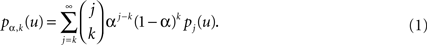We have investigated the distribution of the extinction time of SBP depending on the vaccination level α. To this end, for each α such that 0 ≤ α ≤ 1, we denote by Tα the time to extinction of SBP initiated at time 0 with a single infected individual, with family of infection distribution laws {pα,k(u)}k≥0, u > 0, and with distribution function (d.f.) of the survival time G(·). Intuitively, Tα is the maximal time that the infection survives into the population when the proportion of immune individuals is α. Let us denote by

vα(t) = P(Tαt),

its distribution function. Let m(u) be the mean of contacts of an infected individual with survival time u and mα(u) be the mean of susceptible individuals which are infected by a contagious individual with survival time u, given that the proportion of immune individuals in the population is α. Let also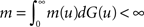and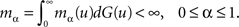Then, from Eq. 1 it is easy to calculate that mα = (1 − α)m.

Moreover, let qα be the extinction probability of a SBP with family of reproduction laws {pα,k(u)}k≥0, u > 0. It is clear that qα = P(Tα < ∞) and is also well-known that qα = 1 if mα ≤ 1 (see Sevast’yanov, 1971). Notice that mα is the critical threshold parameter of our model, that is the basic reproduction number, R0 (or R0,α).

We denote by αinf = max{0,1 − m−1} the smallest proportion of immune individuals, so that the infectious disease becomes extinct almost surely.

To develop the vaccination policies for determining the optimal vaccination rate, we have studied (see González et al., 2010b) the stochastic continuity and monotony properties of the distribution function vα(·). We have proved that vα(·) is monotonically non-decreasing with α, which intuitively means that the greater the proportion of immune individuals, the more probable that the infectious disease will disappear faster. Also we have obtained that the d.f. vα(·) is continuous depending on α, for any α in αinf ≤ α ≤ 1. Continuity and monotonicity properties of the mean time to extinction and of the quantiles of the distribution of the infection extinction time, depending on the vaccination rate α have been derived from that.

## Vaccination Policies

### Based on the Mean of the Time to Extinction

For fixed τ > 0, we are interested in vaccination rates, which guarantee that the average time to extinction of an infection after vaccination period (ended at time, namely, t1) is less than or equal to τ. We determine these vaccination policies as follows. Let us suppose that we have vaccinated a proportion α of susceptible individuals and that at time t1 there were z infected individuals. If we denote by μα,z the mean of the time to extinction of an infectious disease initiated with z infected individuals when the proportion of immune individuals in the population is α, then any vaccination level α such that μα,z ≤ τ could be followed. The optimal vaccination policy is that one which corresponds to the smallest α of all of them, i.e.,

αμ = αμ(τ,z) = inf{α:αinf ≤ α ≤ 1,μα,z ≤ τ}.

### Based on the Quantiles of the Time to Extinction

For fixed p and τ, with 0 < p < 1 and τ > 0, we look for αs, which guarantee that the infectious disease becomes extinct, with probability greater than or equal to p, not later than time τ after the vaccination process ended. If there are z infected individuals at time t1, then the probability that the disease becomes extinct no later than time t1 + τ is equal to vα,z(τ) = (vα(τ))z, with vα,z(·) denoting the distribution function of the time to extinction when the process starts with z infected individuals and α is the proportion of immune individuals in the population.

We define the optimal vaccination policy by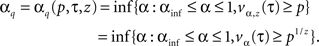For both methods, a deep discussion on how to estimate z and other questions related with this can be found in González et al. (2010a). Moreover, in order to determine optimal α, we approximate vα(·) by means of Monte-Carlo method (see Martìnez and Slavtchova-Bojkova, 2005). Finally, notice that these optimal values are attained due to the monotonicity and continuity properties of vα(·) and μα,z exposed in Section “Branching Model of Epidemic Spread”.

## Comparison of Vaccination Policies Based on Simulations

In the previous section we have proposed two vaccination policies. That gives rise to the natural question which one and when is reasonably to use? That is why, in what follows we compare the two approaches by way of simulation examples, modeling the spread of the disease by means of SBP with the distributions of the incubation period and of the number of contacts (remain, every contact produces infection when there are no immune individuals in the population) belonging to probability distributions commonly used in epidemic modeling for such situations.

Namely, we consider as incubation period distribution (plus the negligible short contact period) a gamma distribution and for the contact distribution a Poisson distribution with parameter λu, being λ,u > 0. These types of distributions turned out to be appropriate for the incubation period and the number of contacts (or infected individuals generated by one infected individual), respectively (see for example Daley and Gani, 1999; Farrington and Grant, 1999; Mode and Sleemam, 2000; Farrington et al., 2003). Intuitively, λ represents the power of the virus and u the length of the incubation period. Hence, the average number of infected individuals by one infected individual is considered proportional to its incubation period, i.e., the larger incubation period is, the larger will be the number of infected individuals. With respect to incubation distribution, we have chosen gamma distribution with mean 15 and shape 30, which guarantee that the survival period in more than 95% of individuals is between 10 and 21 days. Moreover, with respect to contact distribution we have selected λ = 1/3. A similar model was used to fit H5N1 Vietnam data (see OIE, 2007; González et al., 2010b). For the last selected parameters, we deduce that m, the average number of individuals which are infected by one infectious individual, is 5 (when there are no immune individuals in the population). Moreover, we deduce that αinf = 0.8. This means that to get the disease under control, i.e., to guarantee that it will disappear, we must vaccinate at least 80% of the susceptible individuals. But we want to guarantee not only the extinction, but also that it happens in a given period of time.

To this aim, from now on, we consider that z = 1. Intuitively, this could mean that new outbreaks, after vaccination, start with only one infectious individual. Therefore, in this case, to determine both vaccination policies, we obtain the empirical approximation to the distribution vα(·), for 0.8 ≤ α ≤ 1, using the Monte-Carlo method. To this end, for each α in a grid of step 0.01, 10000 processes have been simulated and their duration have been obtained. As an example, in left graphic of Figure 1 we show the histogram of simulated times to extinction for α = 0.89.

FIGURE 1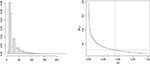Figure 1. Left: Histogram of simulated extinction times for α = 0. 89. Right: Behavior of μα,1 depending on α.

As an illustration of both vaccination policies we take τ = 30, which is actually twice the mean incubation period. In right graphic of Figure 1, the behavior of the mean time to extinction, μα,1, depending on α is shown. Then, we derive that the optimal vaccination policy based on the mean of the time to extinction is αμ(30,1) = 0.89. From the simulated extinction times for α = 0.89 we estimate v0.89(30) by 0.682. This means that if 89% of the population is immunized, then the probability that the disease disappears in less than 30 days is, 0.682. Comparing that to the optimal vaccination policy based on the quantiles, what we are telling is that αq(0.682,30,1) = 0.89. We notice that p = 0.682 is greater than 0.5, because of the skewness of the distribution of the time to extinction (see left graphic of Figure 1). Therefore, vaccinating 89% of susceptible individuals, it is guaranteed that at least 68.2% of new outbreaks take no more than 30 days to disappear. Finally, we notice that this probability is not very high. The larger that probability is, the larger will be the optimal vaccination coverage based on the quantiles. Indeed, in Figure 2, the behavior of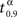, a value such that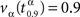, depending on α is shown.is a such value which allows us to establish that 90% of outbreaks, when the proportion of immune individuals in the population is α, will last less than time. From Figure 2, we derive that the optimal vaccination policy based on the quantiles of the time to extinction when p = 0.9 is αq(0.9,30,1) = 0.97, greater than 0.89. Therefore, if we want to guarantee with probability 0.9 that the disease disappears before 30 days, then we have to vaccinate 97% of the susceptible population.

FIGURE 2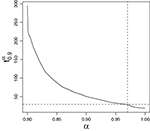Figure 2. Behavior of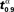depending on α.

From the previous study, we suggest that if the infectious disease is not extremely detrimental for the population and we want to control it in a reasonable time, then the policies based on the mean could be adequate, guaranteeing with probability higher than 0.5, the disease becomes extinct in the desired period of time and therefore it is under control. On the other hand, when the infectious disease is highly detrimental, we would like to eliminate it in the predefined time with high probability. In this case, vaccination policies based on the quantiles are preferable, although this will imply an optimal vaccination rate greater than that based on the mean.

## Discussion

In this review paper we have surveyed two methods for defining an optimal vaccination rate of a population, where a detrimental disease starts to spread. We have tackled this problem using continuous-time branching models, in terms of which then, we have supposed that the age and reproduction of an individual are not necessarily independent. The latter in terms of epidemic takes into account that the number of contacts of an infected individual can depend on the incubation period of the infection. The novelty of our approach is in the use of models allowing us to work in continuous time, as it is in fact in most real world situations. The methods are rather different from the well-established discrete settings, widely used for modeling the early stages of epidemic spread. Concretely, we have used the Bellman–Harris and Sevast’yanov branching processes. These are particular cases of the general branching process which is the model that best fits an epidemic process as it was proved by Ball and Donnelly (1995). Nevertheless, this process is more complicated than both models we have considered, involving more unknown parameters, and our processes are appropriate enough at least to model infectious diseases with incubation period and negligible short contact period. In any case, generalizations of our results in the framework of the general branching processes seem to be an interesting direction for further investigations.

## Conflict of Interest Statement

The authors declare that the research was conducted in the absence of any commercial or financial relationships that could be construed as a potential conflict of interest.

## Acknowledgments

The research was partially supported by the Ministerio de Ciencia e Innovación and the FEDER through the Plan Nacional de Investigación Cientìfica, Desarrollo e Innovación Tecnológica, grant MTM2009-13248 and by appropriated state funds for research allocated to Sofia University (contract No 112/2010), Bulgaria.

## References

Andersson, H., and Britton, T. (2000). Stochastic Epidemic Models and Their Statistical Analysis. Lecture Notes in Statistics, Vol. 151. New York, NY: Springer-Verlag.

Ball, F., and Donnelly, P. (1995). Strong approximations for epidemic models. Stoch. Process. Appl. 55, 1–21.

CrossRef Full Text

Daley, D. J., and Gani, J. (1999). Epidemic Modelling: An Introduction. Cambridge: Cambridge University Press.

De Serres, G., Gay, N. J., and Farrington, C. P. (2000). Epidemiology of transmissible diseases after elimination. Am. J. Epidemiol. 151, 1039–1048.

Farrington, C. P., Kanaan, M. N., and Gay, N. J. (2003). Branching process models for surveillance of infectious diseases controlled by mass vaccination. Biostatistics 4, 279–295.

Farrington, C., and Grant, A. (1999). The distribution of time to extinction in subcritical branching processes: applications to outbreaks of infectious disease. J. Appl. Probab. 36, 771–779.

CrossRef Full Text

González, M., Martìnez, R., and Slavtchova-Bojkova, M. (2009). Age-dependent branching process as models of infectious diseases. C. R. Acad. Bulg. Sci. 62, 541–550.

González, M., Martìnez, R., and Slavtchova-Bojkova, M. (2010a). Stochastic monotonicity and continuity properties of the extinction time of Bellman–Harris branching processes: an application to epidemic modelling. J. Appl. Probab. 47, 58–71.

CrossRef Full Text

González, M., Martìnez, R., and Slavtchova-Bojkova, M. (2010b). “Time to extinction of infectious diseases through age-dependent branching models,” in Workshop on Branching Processes and Their Applications, Vol. 192, Lecture Notes in Statistics-Proceedings, eds M. González, I. del Puerto, R. Martìnez, M. Molina, M. Mota, A. Ramos (New York, NY: Springer Verlag), 241–256.

Isham, V. (2005). “Stochastic models for epidemics (Chapter 1),” in Celebrating Statistics Papers in Honour of Sir David Cox on His 80th Birthday, eds A. C. Davison, Y. Dodge, and N. Wermuth (Oxford: Oxford University Press), 27–54.

Jagers, P. (1975). Branching Processes with Biological Applications. New York, NY: John Wiley and Sons, Inc.

Martìnez, R., and Slavtchova-Bojkova, M. (2005). Comparison between numerical and simulation methods for age-dependent branching models with immigration. Pliska Stud. Math. Bulg. 17, 147–154.

Mode, C. J., and Sleemam, C. K. (2000). Stochastic Processes in Epidemiology. Singapore: World Scientific.

OIE. (2007). Report Reference 1828/TY-DT. World Organization for Animal Health. http://www.oie.int

Pakes, A. (2003). “Biological applications of branching processes (Chapter 18),” Handbook of Statistic, Vol. 21, Stochastic Processes: Modelling and Simulation, eds D. N. Shanbhag and C. R. Rao (Amsterdam: Elsevier Science B.V.), 693–773.

Sevast’yanov, B. (1971). Branching Processes. Moscow: Mir.

Keywords: age-dependent branching process, time to extinction, vaccination policies, Monte-Carlo method

Citation: Slavtchova-Bojkova MN, González M and Martìnez R (2010) Age-dependent branching processes for surveillance of vaccine-preventable diseases with incubation period. Front. Psychiatry 1:127. doi: 10.3389/fpsyt.2010.00127

Received: 03 May 2010; Paper pending published: 21 May 2010;
Accepted: 07 August 2010; Published online: 08 October 2010.

Edited by:

Olcay Akman, Illinois State University, USA

Reviewed by:

Hannah Callender, University of Portland, USA
Necmettin Yildirim, New College of Florida, USA
Gergely Röst, Hungarian Academy of Sciences, Hungary

Copyright: © 2010 Slavtchova-Bojkova, González and Martìnez. This is an open-access article subject to an exclusive license agreement between the authors and the Frontiers Research Foundation, which permits unrestricted use, distribution, and reproduction in any medium, provided the original authors and source are credited.

*Correspondence: Maroussia N. Slavtchova-Bojkova, Faculty of Mathematics and Informatics, Sofia University, No. 5, J. Bourchier Boulevard, 1164 Sofia, Bulgaria. e-mail: bojkova@fmi.uni-sofia.bg